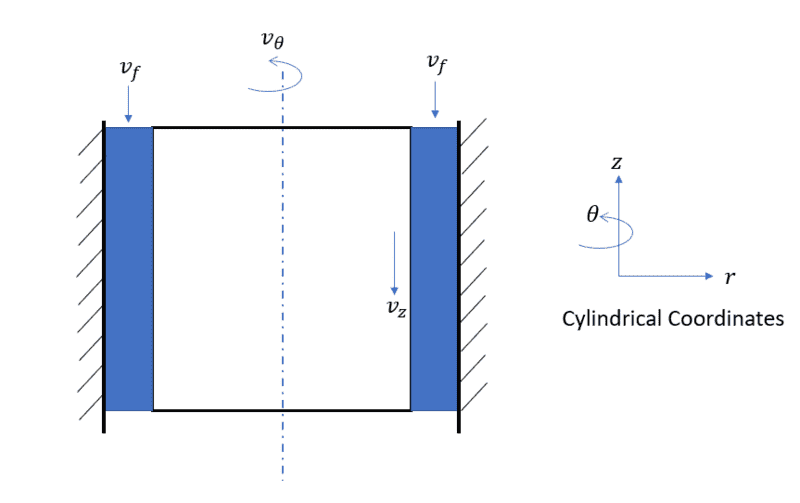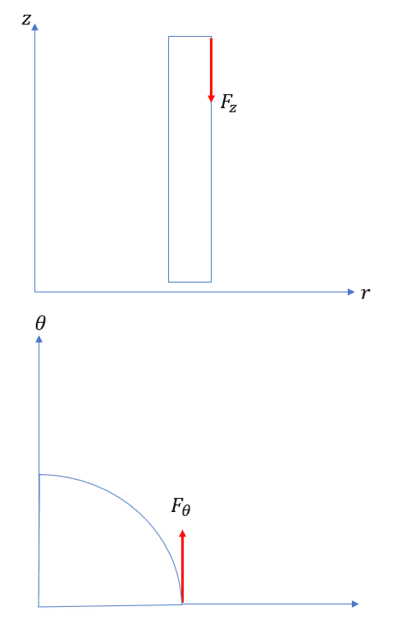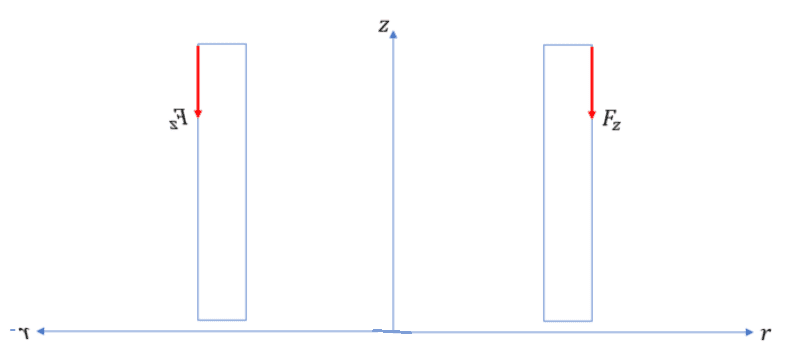# How can I add Moments / Torques in different planes?

• vish0096
vish0096
I have a problem as shown in the figureSo assume there is a cylinder rotating inside a stationally wall. There is a viscous fluid in between the gap of cylinder wall and outer stationary wall, similar to bearing.
The fluid has some velocity in axial direction also
the outer wall of cylinder has velocity in the downward direction and
Moreover the cylinder is rotating about its axis so it will have a circumferential velocity as well.

Now I actually want to calculate the torque required to rotate this cylinder.
I have calculated the circumferetial and axial velocity
Then from that I have calculated the axial and circumferential component of torque, let say T_theta and T_z
Now how can I calculate the resultant torque?
Problem is these two torque act in two different planes, so I am not understanding how can I add them.
The shear forces causeing the torque are shown in the figure belowonatirec
Torque, by definition, is a rotational/circumferential force.

There is no such thing as axial torque; this is just called (linear) force.

Generally, these two components (forces and moments) are kept separate.

You could very well combine them at the surface of the cylinder into a resultant force (not moment/torque); then the circumferential component would implicitly be understood to be a moment (or torque) because of its distance from the axis of rotation.

Edit: Just to go a bit further. T=Fxd. You can divide the total (circumferential) torque by the radius to get the force and simply combine this circumferential force with the axial force using the pythagorean theorem (since they are perpendicular) to get resultant force at the cylinder surface...

•Lnewqban
Homework Helper
2022 Award
There is no such thing as axial torque; this is just called (linear) force.
There are these things called axial vectors for just this circumstance. They are very useful and perhaps you should study them before any more pedagogy.

onatirec
I've gone back and edited this post since my tone was unpleasant. Hutch, I don't appreciate the condescension.

It appears from the problem statement that the axial viscous drag force is balanced on either side, hence the net torque from the axial velocity is zero. OP you left out the axial drag force from the other side of the cylinder.As such, it makes no sense to sum torques (as the T_z would be zero, unless there is some unstated imbalance between the two sides). It would be more appropriate to decompose the loads into forces and combine them as per my first response.

Since this balance of forces from the axial velocity seems immediately apparent to me, I misunderstood a piece of OPs question - thinking they were characterizing the surface force as a torque.

Last edited:
•vish0096 and Lnewqban
Homework Helper
Gold Member
Welcome, vish0096!

I agree with @onatirec

I would try this problem like a screw, calculating the "pitch" by relative velocities and the total resistance to be turned and pushed forward.

•vish0096
vish0096
Thank you, everyone, for your inputs. I am a bit confused about what to do...

So I tend to agree with you that forces because of axial velocity might be balanced.

But I think If I only consider torque due to circumferential force then I might be overestimating the value.

Because if you think of a screw with threads and a screw without threads then off course screw with threads will be much easier to rotate.

So it means axial velocity is actually helping me to reduce required torque so how can I neglect it?

Homework Helper
2022 Award
We suppose the internal drag is laminar (drag ##\alpha~ v##) then $$\vec F_{drag}=-\alpha( \vec v_{axial}+\vec v_{circumferential})$$ where ##\alpha## depends upon contact area and dynamic viscosity. Then $$drag~Torque =-\alpha R v_{circumferential}$$ and $$axial~drag~Force=-\alpha v_{axial}$$ where R is the radius of the cylinder. So the axial speed neither helps nor hurts. Notice if the flow is turbulent, the drag is quadratic, and any additional speed will hurt.

•vish0096
vish0096
Oh great! That made it very clear thanks!

Mentor
First you say that the outer wall is stationary. Then you say that the outer wall has an axial velocity. Which is it.

I would like to see your fluid dynamics analysis of this system. I have experience in this area. To get the torque, all you need to know is the r##\theta## shear stress at either the inner cylinder or the outer cylinder.